# The Earth’s Magnetic Field is Still Losing Energy

## ABSTRACTS

#### D. Russell Humphreys

This paper closes a loophole in the case for a young earth based on the loss of energy from various parts of the earth’s magnetic field. Using ambiguous 1967 data, evolutionists had claimed that energy gains in minor (“non-dipole”) parts compensate for the energy loss from the main (“dipole”) part. However, nobody seems to have checked that claim with newer, more accurate data. Using data from the International Geomagnetic Reference Field (IGRF) I show that from 1970 to 2000, the dipole part of the field steadily lost 235 ± 5 billion megajoules of energy, while the non-dipole part gained only 129 ± 8 billion megajoules. Over that 30-year period, the net loss of energy from all observable parts of the field was 1.41 ± 0.16 %. At that rate, the field would lose half its energy every 1465 ± 166 years. Combined with my 1990 theory explaining reversals of polarity during the Genesis Flood and intensity fluctuations after that, these new data support the creationist model: the field has rapidly and continuously lost energy ever since God created it about 6,000 years ago.

### The Earth’s Magnetic Field is Still Losing Energy

1. INTRODUCTION

Seven centuries ago, a French military engineer, Pierre de Maricourt, carved a sphere out of lodestone, which contains strongly magnetized iron oxide. Using iron needles, he traced the magnetic lines of force around the sphere. He noticed that the lines of force converged upon two points diametrically opposite each other on the sphere. In a widely circulated letter under the name Petrus Peregrinus (1269), he called these points the magnetic poles. Figure 1a shows the magnetic lines of force outside a magnetized sphere. The lines of force outside the sphere have a mathematically precise shape called a dipole field. Having two poles, one north and one south, it has the same shape as the field from a tiny but powerful bar magnet right at the center of the sphere. Another kind of source for a dipole field would be a doughnut-shaped flow of electric current within the sphere, as Figure 1b shows.

Figure 1a. Dipole field around a magnetized sphere. For a purely dipolar field, the equation r = R sin2q relates the radius r and colatitude q of each point on a given line of force, R being the value of r where the line of force intersects the equatorial plane.

 Figure 1b.Westward electric current in the earth’s core which would generate a purely dipolar magnetic field. The oval lines are contours of constant current density (amperes per square meter). Current is high in the bright regions, low in the dark regions. Contours calculated from Barnes’ solution for current density (Barnes, 1973, p. 228, eq. 57).

Three centuries later, William Gilbert (1600), Queen Elizabeth’s personal physician, carefully compared observations of the earth’s magnetic field with the field of a lodestone sphere. He found them very similar. The field of the earth is indeed close to being that of a dipole, though the dipole’s axis tilts about 11.5° away from the earth’s rotational axis. However, the actual field in some places can deviate from that of a purely dipole field by as much as 10% in direction and intensity. Early in the nineteenth century, Carl Friedrich Gauss (1833; 1839) used many measurements from all over the world to characterize the earth’s field. Using what is now called “spherical harmonic analysis,” he mathematically divided the field into dipole and non-dipole parts. The non-dipole parts of the earth’s field have more than two poles. For example, the quadrupole part has a four-pole shape, such as a square of four bar magnets would produce (Figure 2b). A cube of bar magnets, having eight corners and eight poles, would produce an octopole field (Figure 2c), and so forth in multiples of two. One name for each part of the field is harmonic. Another is “mode.”

Figure 2. Dipole and non-dipole magnetic fields from bar magnets: (a) dipole, (b) quadrupole, and (c) octopole. Each source can have various orientations relative to the coordinate axes. The actual sources of the fields in the earth’s core are various distributions of electric current.

Of course, the actual cause of the earth’s non-dipole field is not bar magnets, but simply small irregularities in the electric current in the earth’s core. For example, suppose the doughnut-shaped flow of current I mentioned above were not lined up exactly with the earth’s center, but offset a bit northward above the center. Then the resulting field would have most of the non-dipole parts we observe in the earth’s field (Benton and Alldredge, 1987). The strength of the source of each part of the field is called its moment, such as the “dipole moment” and the “quadrupole moment.” Gauss found that the earth’s magnetic dipole moment is an order of magnitude stronger than any of the non-dipole moments. Scientists after Gauss continued to make global measurements of the field. Three decades ago, Keith McDonald and Robert Gunst (1967; 1968) published the first systematic analysis of such measurements, covering the whole period from 1835 to 1965. They drew a startling conclusion: during those 130 years, the earth’s magnetic dipole moment had steadily decreased by over eight percent! Such a fast change is astonishing for something as big as a planetary magnetic field. Nevertheless, the rapid decline remained relatively unknown to the public, a “trade secret” known mainly to researchers and students of geomagnetism.

2. THE GEOMAGNETIC WARS

3. The International Geomagnetic Reference Field

First, we need more accurate data than what was available in 1967. Figure 3 shows why. This figure reproduces the McDonald and Gunst figure [1967, p. 28, Figure 3(e)] on which Dalrymple based his claim. It shows a curve depicting the “mantle” energy (from the top of the core to the surface) as first decreasing and then increasing. However, the data for the latter part of the curve have a lot of scatter, deviating widely from the curve. For example, in 1965, two points are 1.2 and 1.6% below the curve, while the two others are 1.6 and 6.4% above the curve. A data spread of 8%, four times greater than the 2% upswing the curve alleges, should not give anyone great confidence in the trend.

Figure 3. Reproduction of Figure 3(e) from McDonald and Gunst (1967, p. 28), showing “Total poloidal field energy in mantle,” which is the total observable magnetic field energy between the top of the earth’s core and the earth’s surface, not including the energy above the surface. In their graph each energy unit, 1024 ergs, corresponds to 1017 joules, or 100 petajoules (1 PJ = 1015 joules).

McDonald and Gunst (1967, p. 30) explain the large scatter as being caused by “errors of analysis of higher degree terms. [In extrapolating surface data down to the top of the earth’s core] small errors in the harmonic coefficients are unduly amplified.” They add, “Likewise in Fig. 3(e) we have not been able to enter meaningful information from the analyses of epoch 1965.” In 1968, perhaps in response to the above kinds of issues, the International Association of Geomagnetism and Aeronomy (IAGA) began more systematically measuring, gathering, and analyzing geomagnetic data from all over the world. This group of geomagnetic professionals introduced a “standard spherical harmonic representation” of the field called the International Geomagnetic Reference Field, or IGRF. Every five years, starting in 1970, they have published the dipole moment and higher moments of the field out to the 10th harmonic. Using old data, the IAGA also extended the model back to the year 1900. They now have a standardized set of geomagnetic data spanning the whole twentieth century, 21 epochs of 120 coefficients each. Several journals have concurrently published the most recent version. You can download it free of charge as an ASCII file, a table of over 2500 numbers, from several sites on the Internet (Mandea et al., 2000). One of the Internet sites has an article listing the estimated accuracies, which I have used here (Lowes, 2000). The IGRF is the best set of global geomagnetic data available, accurate enough to give reasonably good values for the non-dipole energies, especially from 1970 until now. Table I shows the data for that period.

4. Calculating the Energy in the Field

In this section, I show how to use the IGRF data to calculate the electrical energy stored in the earth’s magnetic field. If you do not wish to know the mathematical details, just skip to the next section. If you want to study basic electromagnetics, or refresh your memory of it, I recommend Dr. Barnes’s very clear undergraduate textbook, Foundations of Electricity and Magnetism (1965). The magnetic flux intensity B at a location in space tells us how strongly and in what direction the field would compel a compass needle to point. (Bold font denotes a vector, and all quantities are in SI units.) In regions where there is no electric current, which is approximately true outside the earth’s core, we can represent the magnetic flux intensity as the gradient Ñ of a magnetic scalar potential F: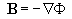The IGRF model gives a spherical harmonic expansion of the magnetic scalar potential for a given date. I define Fn as the component of potential associated with the nth harmonic, so the total magnetic potential becomes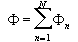The integer n labeling a harmonic is called the degree. Taking the gradient of this equation, we can write the total magnetic flux density as a sum of components: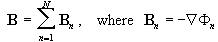The IGRF specifies the nth component of the magnetic potential as a sum of n + 1 terms: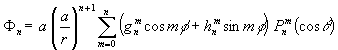Here a is the mean radius of the earth, 6371.2 km; r is the radial distance from the Earth’s center, f is the longitude eastward from Greenwich, q is the geocentric colatitude (90° minus latitude), and   is the associated Legendre function of degree n and order m normalized according to the convention of Schmidt (Merrill and McElhinny, 1983, p. 24). The numbers   and  are called the Gauss coefficients. The IGRF model truncates the expansion at the tenth harmonic, N=10. As many textbooks show, the energy density (joules per cubic meter) stored in the magnetic field B at a given point is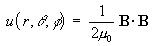The dot represents the scalar product, and 0 is the magnetic permeability of the vacuum (which is essentially the same as the magnetic permeability of the earth). To obtain the total energy E contained in the magnetic field outside the Earth’s core, we must volume-integrate Equation (5) from the radius of the core, = 3471 km, out to infinity: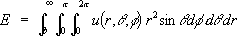Now examine in more detail at the energy density u which goes into this integral. Expanding B in Equation (5) by using the sum in Equation (3a) gives us: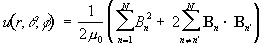In the first summation, Bn is the magnitude of the vector Bn. The second summation contains the cross-terms resulting from squaring the sum in Equation (3a). In doing the angular part of the volume integral of Equation (6), we find that the cross-terms drop out because of the orthogonality of the spherical harmonic functions chosen for Equation (4) (Merrill and McElhinny, 1983, p. 24). That leaves us with a much simpler expression,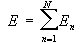where each of the energy components is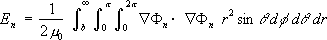Using Equation (4) to expand Equation (9), and using orthogonality to eliminate cross-terms in m, we get the energy En of the nthharmonic in a useful form: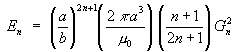where we recall that a and b are the radii of the earth’s surface and core, respectively, and where Gn2 is the sum of the squares of the Gauss coefficients for the nth harmonic: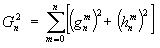McDonald and Gunst [1967, p. 27, Equations (3.7), (3.8)] give this result in a slightly different form. First, to change from their Gaussian units to our SI units, we must replace their relative permeability m  with m 0/4p. Second, we must change their Re to my a, and their e to my a/b. Third, we must add their equations (3.7) and (3.8) to get the total energy for all harmonics. When we sum my Equation (10) over all harmonics as in Equation (8), we get the same result. As another check, the numerical values of my results using IGRF data agree, within five percent, with the graphs of McDonald and Gunst for the period in common having the least scatter, 1915 to 1925. The small disagreement is due to differences of several percent in the Gauss coefficients in the two data sets. The differences arose from different ways of analyzing the raw magnetic data. For example, McDonald and Gunst for practical reasons truncated their analysis with N = 6, whereas the IGRF went out to the tenth harmonic. Since the difference in data accounts for the difference in results satisfactorily, the approximate agreement is further support for equations (10) and (11). The factor a/b in Equation (10) is the ratio of the earth’s surface radius to the radius of the core. Since the equation raises this factor, 1.835, to the power 2+ 1, the higher harmonics have much more weight relative to the lower harmonics. That is why it is very important to secure accurate data for the higher harmonics. Equations (10) and (11) give the total magnetic energy outside the core radius, r=b. Although magnetic fields and energies also exist in the core as well as outside it, observations of the field outside the core cannot determine the field in the core. Different distributions of electric currents, fields, and energy in the core can give the same field outside the core. Furthermore, “toroidal” fields could exist entirely within the core. However, indirect evidence indicates toroidal fields are small, as I mention in section 2. A spherical harmonic expansion of fields inside the core would invert the radial factors, so that they would be of the form (r/b) n + 1[Smythe, 1989, Section 7.12, Equation (5)]. That implies that field intensities in the core should not be drastically different than those at its surface. Since (r/b) ≤ 1 in the core, the lower harmonics should dominate. These considerations suggest that the ratio of non-dipole to dipole energy would not change much if we could somehow include the contribution of fields in the core. Anyway, we can do no better than to use the fields we can measure. Thus, the E of Equation (8) is the total observable energy. Benton and Alldredge [1987, p. 266, Equations (2), (3)] give the energy only above the earth’s surface, r=a. They define Gn2 with a multiplying factor that I have instead placed into the final energy equation. If we put (a/b) = 1 into my equations, the result agrees with theirs. A final caveat is that extrapolating the IGRF model down to the top of the core does not account for electric currents in the mantle and magnetization in the crust. However, the electrical conductivity of the core is much greater than that of the mantle or crust, and evidence suggests that magnetic sources outside the core are relatively small. For example, it appears that crustal magnetization only affects harmonics higher than the tenth (Benton and Alldredge, 1987, p. 271, Figure 2).

5. Results and Accuracy

Table II and Figure 4 show the energies contained in the earth’s magnetic field from the years 1900 to 2000, according to the IGRF data (of which Table I is a sample) and equations (8), (10), and (11). The dipole energy is E1, the non-dipole energy is the sum of E2 through E10, and the total energy E is the sum of E1 through E10. The last row shows Lowes’s (2000) estimates of rms error in Baveraged over the earth’s surface. The rows labeled with sigmas (s) show the corresponding errors in the various calculated energies.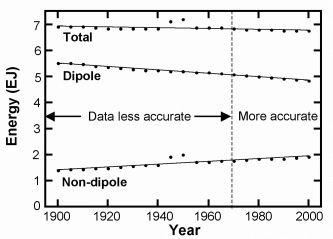Figure 4. Dipole, non-dipole, and total energies computed by Equations (10) and (11) from the International Geomagnetic Reference Field (IGRF) for the entire twentieth century. Energy units are exajoules (1 EJ = 1018 joules = 1000 PJ). From 1970 onward, the non-dipole data are more accurate than for earlier years. Sections 5 and 6 discuss the “pulse” in the non-dipole energy during 1945 and 1950.   Lines are least-squares exponential fits that include the points from those two epochs.

The most important thing to notice in these numbers is the great loss of energy from the field. According to the IGRF data, the total observable energy decreased over 2.6% during the twentieth century. This loss of 180 ± 34 petajoules (1 petajoule = 1 PJ = 1015 joules = 1 billion megajoules) amounts to 50 billion kilowatt-hours of electrical energy - enough to power over five million U.S. households for a year. Notice that the energy loss was steady except during two epochs, 1945 and 1950. Between 1940 and 1950, according to the IGRF model, total energy jumped up by a remarkable + 4.7%. Then from 1950 to 1955, the total energy plummeted even more rapidly by almost the same amount (- 4.2% of the 1940 value), to a value about equal to what the century-averaged trend (curve fit in Figure 4) would give for 1955. In other words, though there may have been a temporary “pulse” of energy that decade, it disappeared and left no lasting impression on the long-term energy trend. Moreover, all of the pulse came from the non-dipole component. Notice that during the period in question, the dipole energy continued its steady decay and suffered no corresponding pulse in the negative direction. However we interpret the pulse, the steady dipole decay contradicts Dalrymple’s conjecture: that increases in non-dipole energy ought to be fed by losses in dipole energy. The pulse of non-dipole energy may not be real. It turns out that essentially all of the pulse comes from a roughly 300% rise and fall in the energy of the 9th and 10th harmonics alone. According to McDonald and Gunst (1967, pp. 29-30), harmonics higher than the 6th had errors large enough during that period to cause problems. As Figure 3 shows, the energy data have a large scatter during that time. Figure 5 shows the estimates I mentioned above by one of the IGRF authors (Lowes, 2000) of the rms errors in Bpredicted by the model during the twentieth century. Notice that there is a large bump in error at the same time as the alleged pulse. This casts more doubt on the reality of the pulse. However, if it is real, I offer a possible explanation in the next section. Because of the efforts of geomagnetists after 1968 to measure and characterize the field more systematically, the data from 1970 to 2000 are much more accurate than the earlier data. That is especially so in years when satellite data added greatly to the precision. Figures 6 and 7 show the dipole and non-dipole energies during the accurate period. The straight lines in the figures are least-squares exponential curve fits. The fits show that during those 30 years the dipole lost 235 ± 5 PJ, whereas the non-dipole part gained only 129 ± 8 PJ. Contrary to Dalrymple’s hope, the sum of the two energies decreased. Figure 8 shows the decline of the total (dipole plus non-dipole) observable energy from 1970 to 2000, again with an exponential curve fit. The fit gives an energy decay time of 2113 ± 239 years, or an energy half-life of 1465 ± 166 years. That means the net loss of energy during the 30-year period was 96 ± 11 PJ. In 30 years, 1.41 ± 0.16 % of all the observable magnetic energy disappeared.

 Figure 5. Estimated root mean square error in the IGRF magnetic field intensity B at the earth’s surface (Lowe, 2000), in nanoteslas.Figure 6. Dipole energy decrease from 1970 through 2000.Figure 7. Non-dipole energy increase from 1970 through 2000. Figure 8. Total energy decrease from 1970 through 2000.

6. Where the Energy Went

As far as we know, natural processes cannot destroy energy, so the energy lost from the observable magnetic field had to go somewhere. Either (1) it went into magnetic fields hidden from our view inside the core, or (2) the processes in the core converted it into some other form of energy. Possibility (1) is unlikely, because several natural processes expel magnetic flux (lines of force) upward out of the core (Humphreys, 1990, p. 131). These are transport, buoyancy, and diffusion of magnetic flux lines. First, according to Alfven’s theorem and observations (Shercliff, 1965), upward flows of the electrically conductive fluid in the core sweep magnetic flux up with them to the surface, as Figure 9(a) shows. Second, magnetic buoyancy (Parker, 1983; Wissink, et al., 2000) tends to prevent flux from going back down with downward flows of the fluid. Third, magnetic diffusion pushes flux upward out of the core into the less conductive mantle rock, Figure 9(b). Thus, flux tends to emerge from the core, not disappear back into it.

Figure 9. A magnetic line of force emerges out of the earth’s core. (a) An upflow of the electrically conductive core fluid pushes a section of the line up to the outer surface of the core, and magnetic buoyancy keeps much of the line from descending with downflows. (b) The line of force diffuses upward out of the core.

My 1990 paper shows that as upwelling core fluid expels magnetic flux, it also generates loops of new lines of force in the reversed direction. If the motions are fast enough to generate new flux lines faster than dissipative processes (discussed below) can destroy them, then eventually the new flux loops can combine and emerge from the core as large loops of flux of reversed direction from the previous field. However, as I showed in the paper, the new flux is never as great as the old flux, because of dissipative processes. Thus, the new reversed (mostly dipole) field could never have as much energy as the previous field. The only demonstrated possible core process I know of which might add energy to a magnetic field is the stretching-out of lines of force (by differential rotation of the fluid) into a “toroidal” east-west direction, as possibly happens on the Sun (Humphreys, 1986, p. 116). The energy for the stretching comes from the motions of the fluid. However, as I pointed out in section 2, observational evidence weighs against strong toroidal fields existing now in the earth’s core. One process we can be certain about is ohmic dissipation or “joule heating.” Because the core is not a perfect electrical conductor, its electrical resistance will continually be eroding the electrical currents in the core, converting magnetic field energy into heat. As I mentioned in section 2, the observed rate of loss of magnetic energy is quite consistent with observed electrical resistivity of likely core materials under core conditions. Thus, the missing field energy is most likely to have become heat in the earth’s core. What about the general increase of non-dipole energy? My model would suggest it is simply due to the motions of the fluid “chopping up” dipole flux lines of force into smaller loops of flux, which will then dissipate their energy faster. McDonald and Gunst (1967, p. 25, italics mine) agree: This [nondipole energy increase] leads us to conclude that the zonal dipole field is being driven destructively to smaller values by fluid motions which transform its magnetic energy into that of the near neighboring higher-order modes [harmonics] rather than expend it more directly as joule heat. The joule heating rate associated with the original dipole energy necessarily increases, however, since the free decay period decreases monotonically with increased degree of mode. In other words, the smaller loops of flux will dissipate their energy as heat even faster than the larger loops do. So presently fluid flows are converting some of the dipole energy to into non-dipole energy. However, rapid ohmic dissipation of the non-dipole energy is continually destroying much of the non-dipole energy even while the fluid flows are generating it. According to both my model and the picture given by McDonald and Gunst, the rate of conversion from dipole to non-dipole energy should be proportional to the amount of dipole flux. For example, if there were no dipole flux, no energy would be added to the non-dipole parts. In the future, when the dipole flux will be weaker, the conversion of dipole to non-dipole energy will slow down. But the ohmic dissipation of non-dipole energy will proceed unabated. At some time, dissipation will exceed production. After that time, even the non-dipole energy will decrease. It is interesting that Dalrymple did not seem to perceive the implications of the McDonald and Gunst quote above. If he had, he would have had less reason to hope for the long-term preservation of magnetic energy. What about the pulse of magnetic energy in the 9th and 10th harmonics in 1945 and 1950? If it was real, it may have been caused by the expulsion of a medium-sized loop of flux completely out of the core into the mantle, as Figure 10 shows. Since the electric currents maintaining such a loop would be entirely within the low-conductivity mantle, the magnetic energy of the loop would dissipate quickly, not contributing to the accumulation of non-dipole energy. However, it may have had some effect on the rotation of the earth’s mantle, perhaps eventually resulting in the “geomagnetic jerk” observed in 1969 (Courtillot and Le Mouël, 1984).

 Figure 10. Medium-sized magnetic lines of force move completely out of the core into the mantle. Possibly such an event caused the non-dipole energy “pulse” of 1945-1950.